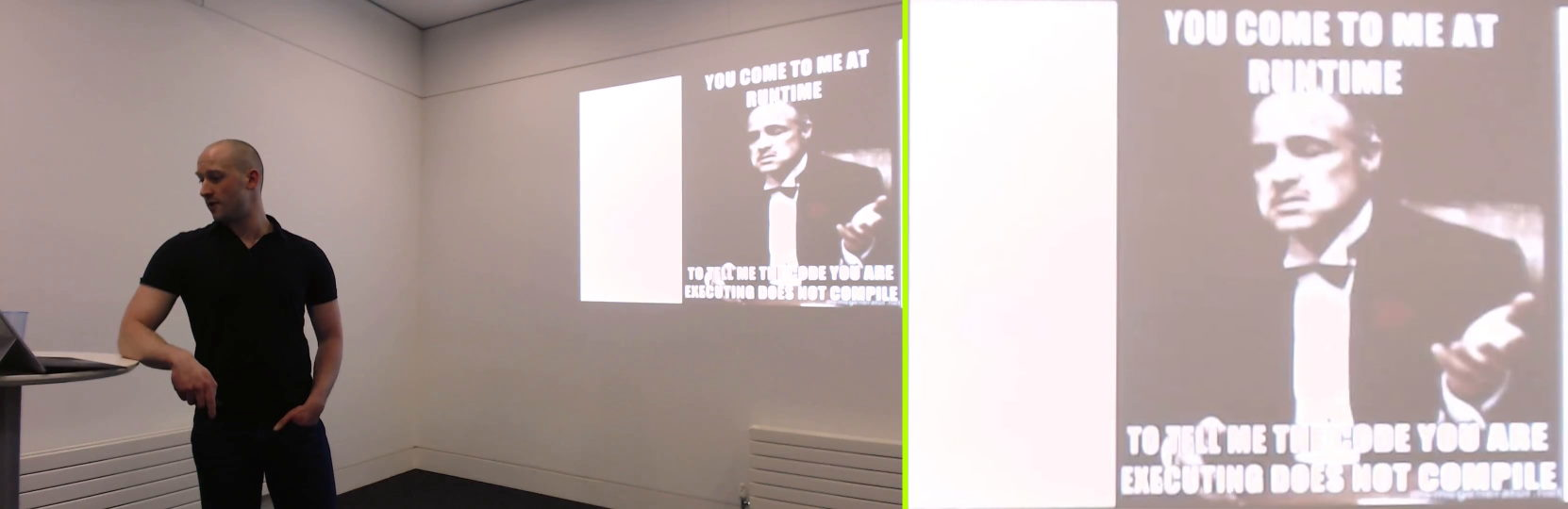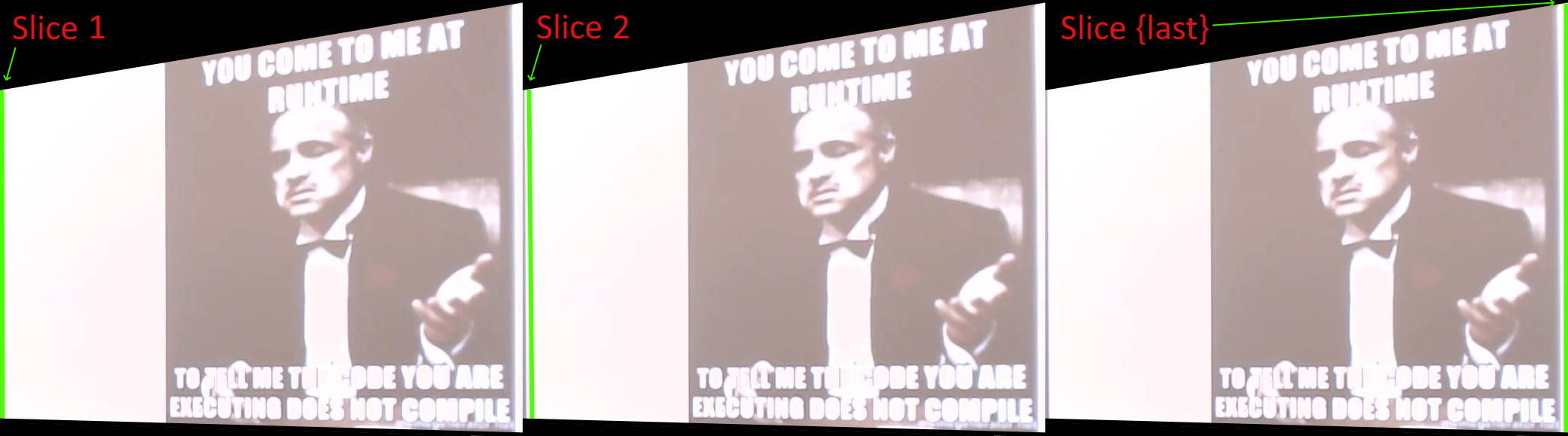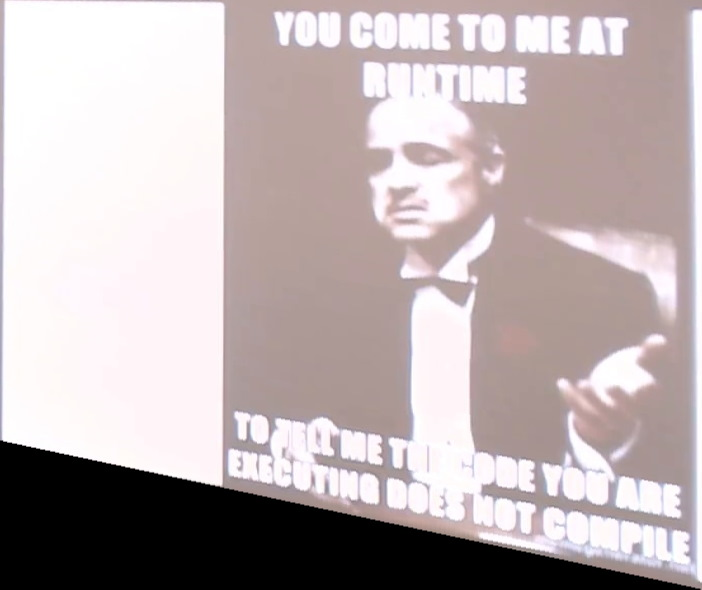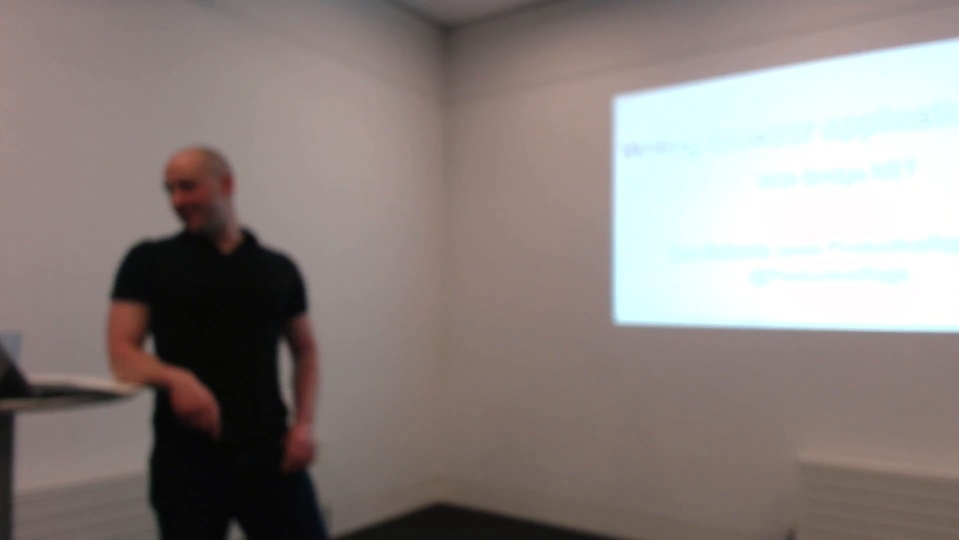# Productive Rage

Dan's techie ramblings

## (Approximately) correcting perspective with C# (fixing a blurry presentation video - part two)

### TL;DR

I have a video of a presentation where the camera keeps losing focus such that the slides are unreadable. I have the original slide deck and I want to fix this.

Step one was identifying the area in each frame that it seemed likely was where the slides were being projected, now step two is to correct the perspective of the projection back into a rectangle to make it easier to perform comparisons against the original slide deck images and try to determine which slide was being projected.

(An experimental TL;DR approach: See this small scale .NET Fiddle demonstration of what I'll be discussing)### The basic approach

An overview of the processing to do this looks as follows:

1. Load the original slide image into a `Bitmap`
2. Using the projected-slide-area region calculated in step one..
1. Take the line from the top left of the region to the top right
2. Take the line from the bottom left of the region to the bottom right (note that this line may be a little longer or shorter than the first line)
3. Create vertical slices of the image by stepping through the first line (the one across the top), connecting each pixel to a pixel on the bottom line
3. These vertical slices will not all be the same height and so they'll need to be adjusted to a consistent size (the further from the camera that a vertical slice of the projection is, the smaller it will be)
4. The height-adjusted vertical slices are then combined into a single rectangle, which will result in an approximation of a perspective-corrected version of the projection of the slide

Note: The reason that this process is only going to be an approximation is due to the way that the height of the output image will be determined -

1. For my purposes, it will be fine to use the largest of the top-left-to-bottom-left length (ie. the left-hand edge of the projection) and the top-right-to-bottom-right length (the right-hand edge of the projected) but this will always result in an output whose aspect ratio is stretched vertically slightly because the largest of those two lengths will be "magnified" somewhat due to the perspective effect
2. What might seem like an obvious improvement would be to take an average of the left-hand-edge-height and the right-hand-edge-height but I decided not to do this because I would be losing some fidelity from the vertical slices that would be shrunken down to match this average and because this would still be an approximation as..
3. The correct way to determine the appropriate aspect ratio for the perspective-corrected image is to use some clever maths to try to determine that angle of the wall that the projection is on (look up perspective correction and vanishing points if you're really curious!) and to use that to decide what ratio of the left-hand-edge-height and the right-hand-edge-height to use
• (The reason that the take-an-average approach is still an approximation is that perspective makes the larger edge grow more quickly than the smaller edge shrinks, so this calculation would still skew towards a vertically-stretched image)

### Slice & dice!

So if we follow the plan above then we'll generate a list of vertical slices a bit like this:.. which, when combined would look like this:This is very similar to the original projection except that:

• The top edge is now across the top of the rectangular area
• The bottom left corner is aligned with the left-hand side of the image
• The bottom right corner is aligned with the right-hand side of the image

We're not done yet but this has brought things much closer!

In fact, all that is needed is to stretch those vertical slices so that they are all the same length and; ta-da!### Implementation for slicing and stretching

So, from previous analysis, I know that the bounding area for the projection of the slide in the frames of my video is:

``````topLeft: (1224, 197)
topRight: (1915, 72)

bottomLeft: (1229, 638)
bottomRight: (1915, 662)
``````

Since I'm going to walk along the top edge and create vertical slices from that, I'm going to need the length of that edge - which is easy enough with some Pythagoras:

``````private static int LengthOfLine((PointF From, PointF To) line)
{
var deltaX = line.To.X - line.From.X;
var deltaY = line.To.Y - line.From.Y;
return (int)Math.Round(Math.Sqrt((deltaX * deltaX) + (deltaY * deltaY)));
}
``````

So although it's only 691px horizontally from the top left to the top right (1915 - 1224), the actual length of that edge is 702px (because it's not a line that angles up slightly rather than being a flat horizontal one).

This edge length determines how many vertical slices that we'll take and we'll get them by looping across this top edge, working out where the corresponding point on the bottom edge should be and joining them together into a line; one vertical slice. Each time that the loop increments, the current point on the top edge is going to move slightly to the right and even more slightly upwards while each corresponding point on the bottom edge will also move slightly to the right but it will move slightly down as the projection on the wall gets closer and closer to the camera.

One way to get all of these vertical slice lines is a method such as the following:

``````private sealed record ProjectionDetails(
Size ProjectionSize,
IEnumerable<((PointF From, PointF To) Line, int Index)> VerticalSlices
);

private static ProjectionDetails GetProjectionDetails(
Point topLeft,
Point topRight,
Point bottomRight,
Point bottomLeft)
{
var topEdge = (From: topLeft, To: topRight);
var bottomEdge = (From: bottomLeft, To: bottomRight);
var lengthOfEdgeToStartFrom = LengthOfLine(topEdge);
var dimensions = new Size(
width: lengthOfEdgeToStartFrom,
height: Math.Max(
LengthOfLine((topLeft, bottomLeft)),
LengthOfLine((topRight, bottomRight))
)
);
return new ProjectionDetails(dimensions, GetVerticalSlices());

IEnumerable<((PointF From, PointF To) Line, int Index)> GetVerticalSlices() =>
Enumerable
.Range(0, lengthOfEdgeToStartFrom)
.Select(i =>
{
var fractionOfProgressAlongPrimaryEdge = (float)i / lengthOfEdgeToStartFrom;
return (
Line: (
GetPointAlongLine(topEdge, fractionOfProgressAlongPrimaryEdge),
GetPointAlongLine(bottomEdge, fractionOfProgressAlongPrimaryEdge)
),
Index: i
);
});
}
``````

This returns the dimensions of the final perspective-corrected projection (which is as wide as the top edge is long and which is as high as the greater of the left-hand edge's length and the right-hand edge's length) as well as an `IEnumerable` of the start and end points for each slice that we'll be taking.

The dimensions are going to allow us to create a bitmap that we'll paste the slices into when we're ready - but, before that, we need to determine pixel values for every point on every vertical slice. As the horizontal distance across the top edge is 691px and the vertical distance is 125px but its actual length is 702px, each time we move one along in that 702px loop the starting point for the vertical slice will move (691 / 702) = 0.98px across and (125 / 702) = 0.18px up. So almost all of these vertical slices are going to have start and end points that are not whole pixel values - and the same applies to each point on that vertical slice. This means that we're going to have to take average colour values for when we're dealing with fractional pixel locations.

For example, if we're at the point (1309.5, 381.5) and the colours at (1309, 381), (1310, 381), (1309, 382), (1310, 382) are all white then the averaging is really easy - the "averaged" colour is white! If we're at the point (1446.5, 431.5) and the colours at (1446, 431), (1447, 431), (1446, 432), (1447, 432) are #BCA6A9, #B1989C, #BCA6A9, #B1989C then it's also not too complicated - because (1446.5, 431.5) is at the precise midpoint between all four points then we can take a really simple average by adding all four R values together, all four G values together, all four B values together and diving them by 4 to get a combined result. It gets a little more complicated where it's not 0.5 of a pixel and it's slightly more to the left or to the right and/or to the top or to the bottom - eg. (1446.1, 431.9) would get more of its averaged colour from the pixels on the left than on the right (as 1446.1 is only just past 1446) while it would get more of its averaged colour from the pixels on the bottom than the top (as 431.9 is practically ay 432). On the other hand, on the rare occasion where it is a precise location (with no fractional pixel values), such as (1826, 258), then it's the absolute simplest case because no averaging is required!

``````private static Color GetAverageColour(Bitmap image, PointF point)
{
var (integralX, fractionalX) = GetIntegralAndFractional(point.X);
var x0 = integralX;
var x1 = Math.Min(integralX + 1, image.Width);

var (integralY, fractionalY) = GetIntegralAndFractional(point.Y);
var y0 = integralY;
var y1 = Math.Min(integralY + 1, image.Height);

var (topColour0, topColour1) = GetColours(new Point(x0, y0), new Point(x1, y0));
var (bottomColour0, bottomColour1) = GetColours(new Point(x0, y1), new Point(x1, y1));

return CombineColours(
CombineColours(topColour0, topColour1, fractionalX),
CombineColours(bottomColour0, bottomColour1, fractionalX),
fractionalY
);

(Color c0, Color c1) GetColours(Point p0, Point p1)
{
var c0 = image.GetPixel(p0.X, p0.Y);
var c1 = (p0 == p1) ? c0 : image.GetPixel(p1.X, p1.Y);
return (c0, c1);
}

static (int Integral, float Fractional) GetIntegralAndFractional(float value)
{
var integral = (int)Math.Truncate(value);
var fractional = value - integral;
return (integral, fractional);
}

static Color CombineColours(Color x, Color y, float proportionOfSecondColour)
{
if ((proportionOfSecondColour == 0) || (x == y))
return x;

if (proportionOfSecondColour == 1)
return y;

return Color.FromArgb(
red: CombineComponent(x.R, y.R),
green: CombineComponent(x.G, y.G),
blue: CombineComponent(x.B, y.B),
alpha: CombineComponent(x.A, y.A)
);

int CombineComponent(int x, int y) =>
Math.Min(
(int)Math.Round((x * (1 - proportionOfSecondColour)) + (y * proportionOfSecondColour)),
255
);
}
}
``````

This gives us the capability to split the wonky projection into vertical slices, to loop over each slice and to walk down each slice and get a list of pixel values for each point down that slice. The final piece of the puzzle is that we then need to resize each vertical slice so that they all match the projection height returned from the `GetProjectionDetails` method earlier. Handily, the .NET `Bitmap` drawing code has `DrawImage` functionality that can resize content, so we can:

1. Create a `Bitmap` whose dimensions are those returned from `GetProjectionDetails`
2. Loop over each vertical slice (which is an `IEnumerable` also returned from `GetProjectionDetails`)
3. Create a bitmap just for that slice - that is 1px wide and only as tall as the current vertical slice is long
4. Use `DrawImage` to paste that slice's bitmap onto the full-size projection `Bitmap`

In code:

``````private static void RenderSlice(
Bitmap projectionBitmap,
IEnumerable<Color> pixelsOnLine,
int index)
{
var pixelsOnLineArray = pixelsOnLine.ToArray();

using var slice = new Bitmap(1, pixelsOnLineArray.Length);
for (var j = 0; j < pixelsOnLineArray.Length; j++)
slice.SetPixel(0, j, pixelsOnLineArray[j]);

using var g = Graphics.FromImage(projectionBitmap);
g.DrawImage(
slice,
srcRect: new Rectangle(0, 0, slice.Width, slice.Height),
destRect: new Rectangle(index, 0, 1, projectionBitmap.Height),
srcUnit: GraphicsUnit.Pixel
);
}
``````

### Pulling it all together

If we combine all of this logic together then we end up with a fairly straightforward static class that does all the work - takes a `Bitmap` that is a frame from a video where there is a section that should be extracted and then "perspective-corrected", takes the four points that describe that region and then returns a new `Bitmap` that is the extracted content in a lovely rectangle!

``````/// <summary>
/// This uses a simple algorithm to try to undo the distortion of a rectangle in an image
/// due to perspective - it takes the content of the rectangle and stretches it into a
/// rectangle. This is only a simple approximation and does not guarantee accuracy (in
/// fact, it will result in an image that is slightly vertically stretched such that its
/// aspect ratio will not match the original content and a more thorough approach would
/// be necessary if this is too great an approximation)
/// </summary>
internal static class SimplePerspectiveCorrection
{
public static Bitmap ExtractAndPerspectiveCorrect(
Bitmap image,
Point topLeft,
Point topRight,
Point bottomRight,
Point bottomLeft)
{
var (projectionSize, verticalSlices) =
GetProjectionDetails(topLeft, topRight, bottomRight, bottomLeft);

var projection = new Bitmap(projectionSize.Width, projectionSize.Height);
foreach (var (lineToTrace, index) in verticalSlices)
{
var lengthOfLineToTrace = LengthOfLine(lineToTrace);

var pixelsOnLine = Enumerable
.Range(0, lengthOfLineToTrace)
.Select(j =>
{
var fractionOfProgressAlongLineToTrace = (float)j / lengthOfLineToTrace;
var point = GetPointAlongLine(lineToTrace, fractionOfProgressAlongLineToTrace);
return GetAverageColour(image, point);
});

RenderSlice(projection, pixelsOnLine, index);
}
return projection;

static Color GetAverageColour(Bitmap image, PointF point)
{
var (integralX, fractionalX) = GetIntegralAndFractional(point.X);
var x0 = integralX;
var x1 = Math.Min(integralX + 1, image.Width);

var (integralY, fractionalY) = GetIntegralAndFractional(point.Y);
var y0 = integralY;
var y1 = Math.Min(integralY + 1, image.Height);

var (topColour0, topColour1) = GetColours(new Point(x0, y0), new Point(x1, y0));
var (bottomColour0, bottomColour1) = GetColours(new Point(x0, y1), new Point(x1, y1));

return CombineColours(
CombineColours(topColour0, topColour1, fractionalX),
CombineColours(bottomColour0, bottomColour1, fractionalX),
fractionalY
);

(Color c0, Color c1) GetColours(Point p0, Point p1)
{
var c0 = image.GetPixel(p0.X, p0.Y);
var c1 = (p0 == p1) ? c0 : image.GetPixel(p1.X, p1.Y);
return (c0, c1);
}

static (int Integral, float Fractional) GetIntegralAndFractional(float value)
{
var integral = (int)Math.Truncate(value);
var fractional = value - integral;
return (integral, fractional);
}

static Color CombineColours(Color x, Color y, float proportionOfSecondColour)
{
if ((proportionOfSecondColour == 0) || (x == y))
return x;

if (proportionOfSecondColour == 1)
return y;

return Color.FromArgb(
red: CombineComponent(x.R, y.R),
green: CombineComponent(x.G, y.G),
blue: CombineComponent(x.B, y.B),
alpha: CombineComponent(x.A, y.A)
);

int CombineComponent(int x, int y) =>
Math.Min(
(int)Math.Round(
(x * (1 - proportionOfSecondColour)) +
(y * proportionOfSecondColour)
),
255
);
}
}
}

private sealed record ProjectionDetails(
Size ProjectionSize,
IEnumerable<((PointF From, PointF To) Line, int Index)> VerticalSlices
);

private static ProjectionDetails GetProjectionDetails(
Point topLeft,
Point topRight,
Point bottomRight,
Point bottomLeft)
{
var topEdge = (From: topLeft, To: topRight);
var bottomEdge = (From: bottomLeft, To: bottomRight);
var lengthOfEdgeToStartFrom = LengthOfLine(topEdge);
var dimensions = new Size(
width: lengthOfEdgeToStartFrom,
height: Math.Max(
LengthOfLine((topLeft, bottomLeft)),
LengthOfLine((topRight, bottomRight))
)
);
return new ProjectionDetails(dimensions, GetVerticalSlices());

IEnumerable<((PointF From, PointF To) Line, int Index)> GetVerticalSlices() =>
Enumerable
.Range(0, lengthOfEdgeToStartFrom)
.Select(i =>
{
var fractionOfProgressAlongPrimaryEdge = (float)i / lengthOfEdgeToStartFrom;
return (
Line: (
GetPointAlongLine(topEdge, fractionOfProgressAlongPrimaryEdge),
GetPointAlongLine(bottomEdge, fractionOfProgressAlongPrimaryEdge)
),
Index: i
);
});
}

private static PointF GetPointAlongLine((PointF From, PointF To) line, float fraction)
{
var deltaX = line.To.X - line.From.X;
var deltaY = line.To.Y - line.From.Y;
return new PointF(
(deltaX * fraction) + line.From.X,
(deltaY * fraction) + line.From.Y
);
}

private static int LengthOfLine((PointF From, PointF To) line)
{
var deltaX = line.To.X - line.From.X;
var deltaY = line.To.Y - line.From.Y;
return (int)Math.Round(Math.Sqrt((deltaX * deltaX) + (deltaY * deltaY)));
}

private static void RenderSlice(
Bitmap projectionBitmap,
IEnumerable<Color> pixelsOnLine,
int index)
{
var pixelsOnLineArray = pixelsOnLine.ToArray();

using var slice = new Bitmap(1, pixelsOnLineArray.Length);
for (var j = 0; j < pixelsOnLineArray.Length; j++)
slice.SetPixel(0, j, pixelsOnLineArray[j]);

using var g = Graphics.FromImage(projectionBitmap);
g.DrawImage(
slice,
srcRect: new Rectangle(0, 0, slice.Width, slice.Height),
destRect: new Rectangle(index, 0, 1, projectionBitmap.Height),
srcUnit: GraphicsUnit.Pixel
);
}
}
``````

### Coming next

So step one was to take frames from a video and to work out what the bounds were of the area where slides were being projected (and to filter out any intro and outro frames), step two has been to be able to take the bounded area from any slide and project it back into a rectangle to make it easier to match against the original slide images.. step three will be to use these projections to try to guess what slide is being displayed on what frame!

The frame that I've been using as an example throughout this post probably looks like a fairly easy case - big blocks of white or black and not actually too out of focus.. but some of the frames look like this and that's a whole other kettle of fish!Posted at 19:02